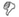Feigenbaum constants love

# Feigenbaum constants

## Definitions

Sorry, no definitions found. You may find more data at feigenbaum constants.

## Etymologies

Sorry, no etymologies found.

## Support## Examples

Sorry, no example sentences found.

• The Feigenbaum constants are two mathematical constants named after the mathematician Mitchell Feigenbaum. Both express ratios in a bifurcation diagram.

The first Feigenbaum constant (sequence A006890 in OEIS),

δ = 4.66920160910299067185320382...

is the limiting ratio of each bifurcation interval to the next, or between the diameters of successive circles on the real axis of the Mandelbrot set. Feigenbaum originally related this number to the period-doubling bifurcations in the logistic map, but also showed it to hold for all one-dimensional maps with a single quadratic maximum. As a consequence of this generality, every chaotic system that corresponds to this description will bifurcate at the same rate. It was discovered in 1975.

The second Feigenbaum constant (sequence A006891 in OEIS),

α = 2.502907875095892822283902873218...,

is the ratio between the width of a tine and the width of one of its two subtines (except the tine closest to the fold).

These numbers apply to a large class of dynamical systems (for example, dripping faucets to population growth).

Both numbers are believed to be transcendental, although they have not been proven to be so.

A proof of the universality of the Feigenbaum constants was given by Mikhail Lyubich in the 1990s.1 -- Wikipedia

to visualize

February 12, 2012